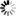Normal view

# Statistical distributions. by Nick T. Thomopoulos

Material type:TextPublisher: New York, NY : Springer Science+Business Media, 2017Description: 172p. 24cmContent type:
• text
Media type:
• unmediated
Carrier type:
• volume
ISBN:
• 9783319651118
Subject(s): DDC classification:
• 519.5 THO
Summary: This book gives a description of the group of statistical distributions that have ample application to studies in statistics and probability. Understanding statistical distributions is fundamental for researchers in almost all disciplines. The informed researcher will select the statistical distribution that best fits the data in the study at hand. Some of the distributions are well known to the general researcher and are in use in a wide variety of ways. Other useful distributions are less understood and are not in common use. The book describes when and how to apply each of the distributions in research studies, with a goal to identify the distribution that best applies to the study. The distributions are for continuous, discrete, and bivariate random variables. In most studies, the parameter values are not known a priori, and sample data is needed to estimate parameter values. In other scenarios, no sample data is available, and the researcher seeks some insight that allows the estimate of the parameter values to be gained. This handbook of statistical distributions provides a working knowledge of applying common and uncommon statistical distributions in research studies. These nineteen distributions are: continuous uniform, exponential, Erlang, gamma, beta, Weibull, normal, lognormal, left-truncated normal, right-truncated normal, triangular, discrete uniform, binomial, geometric, Pascal, Poisson, hyper-geometric, bivariate normal, and bivariate lognormal. Some are from continuous data and others are from discrete and bivariate data. This group of statistical distributions has ample application to studies in statistics and probability and practical use in real situations. Additionally, this book explains computing the cumulative probability of each distribution and estimating the parameter values either with sample data or without sample data. Examples are provided throughout to guide the reader. Accuracy in choosing and applying statistical distributions is particularly imperative for anyone who does statistical and probability analysis, including management scientists, market researchers, engineers, mathematicians, physicists, chemists, economists, social science researchers, and students in many disciplines. Includes 89 examples that help the reader apply the concepts presented Explains how to compute cumulative probability for all distributions including Erlang, gamma, beta, Weibull, normal, and lognormal Utilizes sample data to estimate parameter values of each distribution Estimates parameter values when no sample data Introduces Left-Truncated Normal Introduces Right-Truncated Normal Introduces Spread Ratio.
Tags from this library: No tags from this library for this title.
Star ratingsAverage rating: 0.0 (0 votes)
Holdings
Item type Current library Call number Status Date due BarcodeBook Indian Institute of Management Visakhapatnam - Andhra University 519.5 THO (Browse shelf(Opens below)) Available 001504

This book gives a description of the group of statistical distributions that have ample application to studies in statistics and probability. Understanding statistical distributions is fundamental for researchers in almost all disciplines. The informed researcher will select the statistical distribution that best fits the data in the study at hand. Some of the distributions are well known to the general researcher and are in use in a wide variety of ways. Other useful distributions are less understood and are not in common use. The book describes when and how to apply each of the distributions in research studies, with a goal to identify the distribution that best applies to the study. The distributions are for continuous, discrete, and bivariate random variables. In most studies, the parameter values are not known a priori, and sample data is needed to estimate parameter values. In other scenarios, no sample data is available, and the researcher seeks some insight that allows the estimate of the parameter values to be gained. This handbook of statistical distributions provides a working knowledge of applying common and uncommon statistical distributions in research studies. These nineteen distributions are: continuous uniform, exponential, Erlang, gamma, beta, Weibull, normal, lognormal, left-truncated normal, right-truncated normal, triangular, discrete uniform, binomial, geometric, Pascal, Poisson, hyper-geometric, bivariate normal, and bivariate lognormal. Some are from continuous data and others are from discrete and bivariate data. This group of statistical distributions has ample application to studies in statistics and probability and practical use in real situations. Additionally, this book explains computing the cumulative probability of each distribution and estimating the parameter values either with sample data or without sample data. Examples are provided throughout to guide the reader. Accuracy in choosing and applying statistical distributions is particularly imperative for anyone who does statistical and probability analysis, including management scientists, market researchers, engineers, mathematicians, physicists, chemists, economists, social science researchers, and students in many disciplines. Includes 89 examples that help the reader apply the concepts presented Explains how to compute cumulative probability for all distributions including Erlang, gamma, beta, Weibull, normal, and lognormal Utilizes sample data to estimate parameter values of each distribution Estimates parameter values when no sample data Introduces Left-Truncated Normal Introduces Right-Truncated Normal Introduces Spread Ratio.

There are no comments on this title.